Last updated:

Tested on Ubuntu 20.04

$sudo apt install indicator-multiload  ## Add per-core metrics to indicator To add use percentages for each core, use this example: percent(cpu.cpu0.inuse) • 1) Open Preferences -> Indicator Items • 2) Add the following to the first slot (example for 8 cores): $(percent(cpu.cpu0.inuse)) | $(percent(cpu.cpu1.inuse)) |$(percent(cpu.cpu2.inuse)) | $(percent(cpu.cpu3.inuse)) |$(percent(cpu.cpu4.inuse)) | $(percent(cpu.cpu5.inuse)) |$(percent(cpu.cpu6.inuse)) | $(percent(cpu.cpu7.inuse))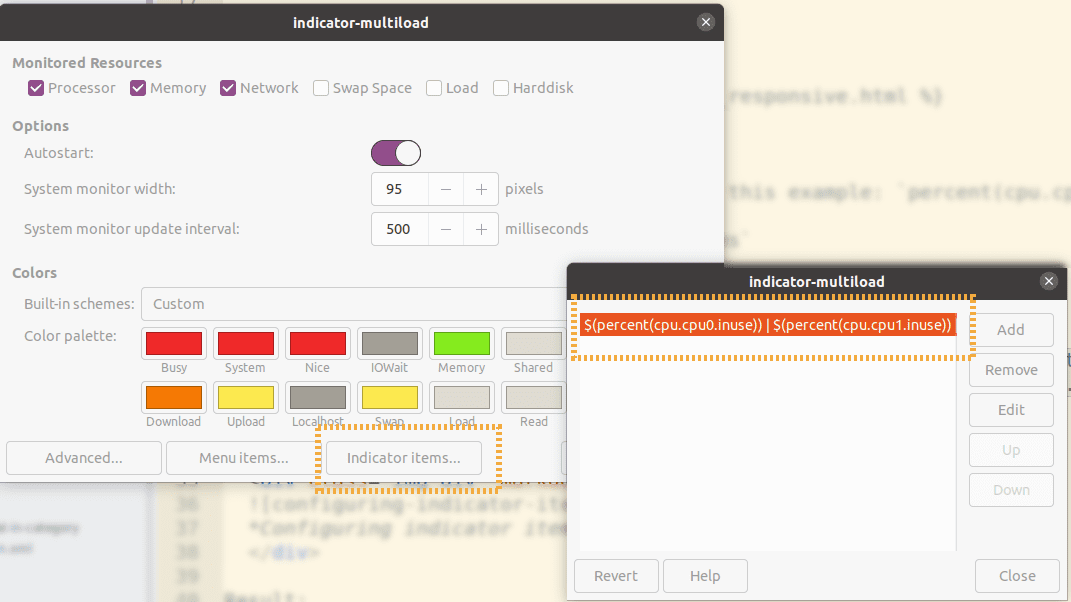Configuring indicator items with percent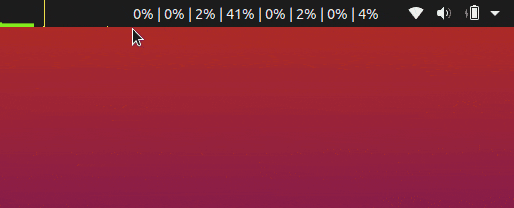RESULT: Added per-core percentage use to the indicator bar (example for 8 cores) ## Add per-core metrics to indicator, fixed width It's annoying that the numbers keep moving depending on how much use you have. To have fixed-width columns, use decimals(..., 2) instead of percentage: • 1) Open Preferences -> Indicator Items... • 2) Add the following to the first slot (example for 8 cores): $(decimals(cpu.cpu0.inuse,2)) | $(decimals(cpu.cpu1.inuse,2)) |$(decimals(cpu.cpu2.inuse,2)) | $(decimals(cpu.cpu3.inuse,2)) |$(decimals(cpu.cpu4.inuse,2)) | $(decimals(cpu.cpu5.inuse,2)) |$(decimals(cpu.cpu6.inuse,2)) | $(decimals(cpu.cpu7.inuse,2))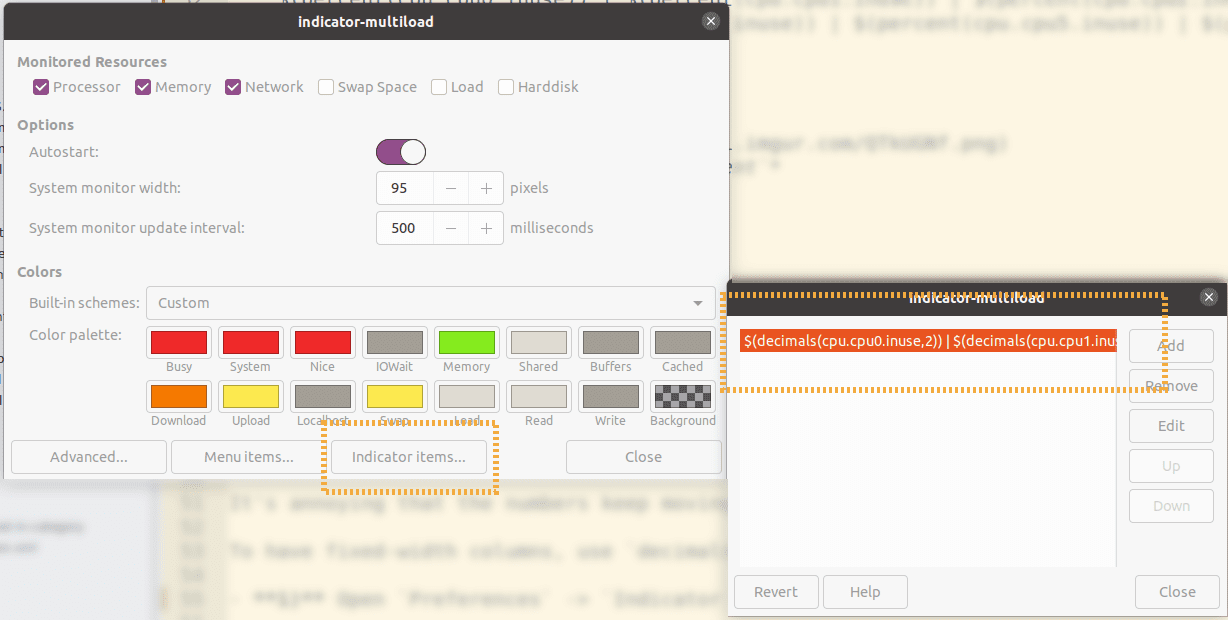use decimals instead of percent to force fixed-width metrics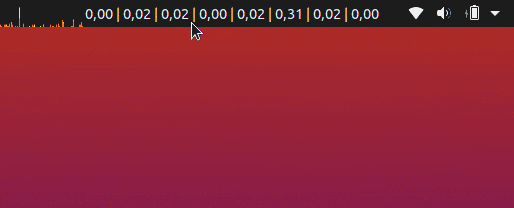RESULT: Slightly different from the above: per-core metrics are now formatted to use 2 decimals, which makes them have a fixed-width (example for 8 cores) ## Add per-core metrics to menu You can also add the per-core metrics to the context menu instead of the indicator launch bar: To have fixed-width columns, use decimals(..., 2) instead of percentage: • 1) Open Preferences -> Menu Items... • 2) Add the following to the first 8 slots (example for 8 cores): CPU0:$(percent(cpu.cpu0.inuse)
CPU1: $(percent(cpu.cpu1.inuse) CPU2:$(percent(cpu.cpu2.inuse)
CPU3: $(percent(cpu.cpu3.inuse) CPU4:$(percent(cpu.cpu4.inuse)
CPU5: $(percent(cpu.cpu5.inuse) CPU6:$(percent(cpu.cpu6.inuse)
CPU7: \$(percent(cpu.cpu7.inuse)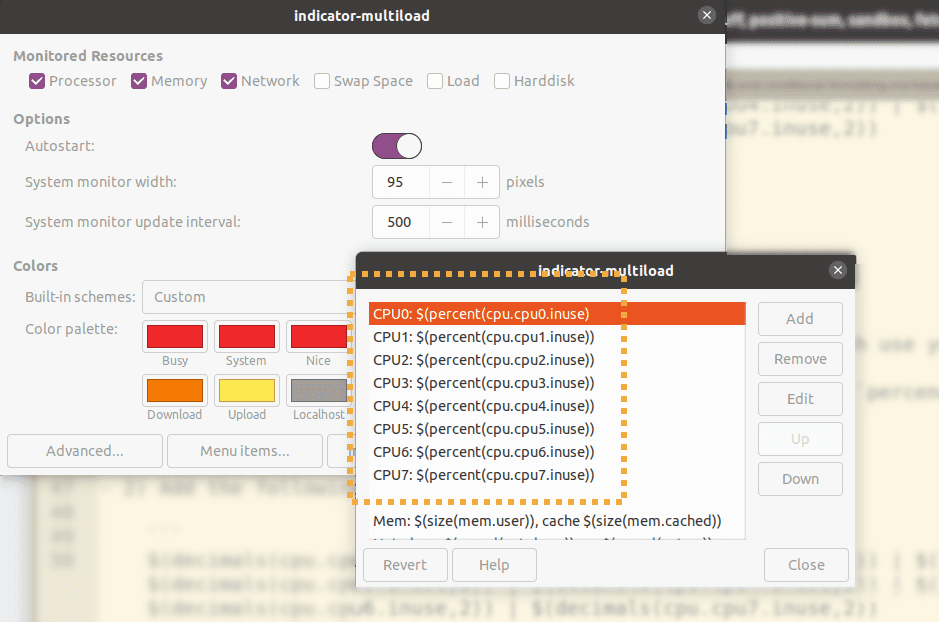Configuring menu items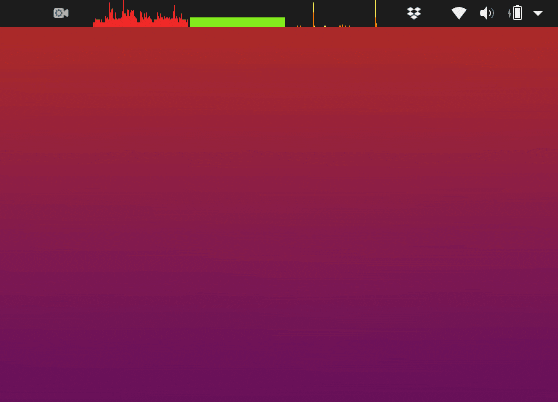RESULT: Per-core CPU usage in the context menu
(example for 8 cores)